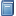Buchempfehlung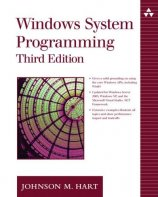Windows System Programming
Das Kompendium liefert viele interessante Informationen zur Windows-Programmierung auf Englisch. [Mehr Infos...]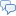FreeBASIC-Chat
Es sind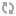Benutzer im FreeBASIC-Chat online.
(Stand:  )

# fb:porticula NoPaste

## Console.bi

 Uploader: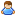OneCypher Datum/Zeit: 13.10.2009 10:58:59
Hinweis: Dieser Quelltext ist Bestandteil des Projekts GuiPtr, zu dem es auf FreeBASIC-Portal.de eine Projektseite gibt.

```#include once "GuiPtr.bi" type TextType     s as string     declare operator cast() as string     declare operator let(v as string)     declare constructor()     declare constructor(v as string) end type constructor TextType() end constructor constructor TextType(v as string)     s = v end constructor operator TextType.Cast() as string     return s end operator operator TextType.let(v as string)     s = v end operator Type Console     Object as GuiObject ptr     declare sub Print(_text as string)     declare sub PrintALine(_text as string)     declare function Locate(r as integer, c as integer) as Console ptr     declare sub cls()     declare sub Color(FColor as integer, BColor as integer)     TextCollection as Collection ptr = new Collection     Rows as integer     Columns as integer     Backcolor as uinteger     BorderStyle as integer     ForeColor as uinteger = RGB(196,196,196)     FontStyle as ubyte     ScrollRow as integer = 1     CursorRow as integer = 1     CursorColumn as integer = 1     declare constructor(l as integer, t as integer, w as integer, h as integer) end type Sub Console.Color(FColor as integer = -1, BColor as integer = -1)     Dim c(0 to 15) as uinteger     C(0) = RGB(0,0,0):    C(1) = RGB(0,0,170)     C(2) = RGB(0,170,0):    C(3) = RGB(0,170,170)     C(4) = RGB(170,0,0):    C(5) = RGB(170,0,170)     C(6) = RGB(170,85,0):    C(7) = RGB(170,170,170)     C(8) = RGB(85,85,85):    C(9) = RGB(85,85,255)     C(10) = RGB(85,255,85):    C(11) = RGB(85,255,255)     C(12) = RGB(255,85,85):    C(13) = RGB(255,85,255)     C(14) = RGB(255,255,85):    C(15) = RGB(255,255,255)     If Fcolor >= 0 then ForeColor = c(FColor)     If Bcolor >= 0 then BackColor = c(BColor) end sub function Console.Locate(r as integer, c as integer) as Console ptr     while r > TextCollection->Count         Print ""     wend     CursorRow = r: CursorColumn = c     return @This end function sub console.cls()     Dim TextPtr as TextType ptr     do         TextPtr = Textcollection->Item(1)         delete TextPtr         TextCollection->Remove(1)     loop until Textcollection->Count = 0     ScrollRow = 1:    CursorRow = 1     CursorColumn = 1 end sub sub Console.Print(_text as string)     Dim SubString as string = _text & CHR(13,10)     Dim SubString2 as string     dim i as integer     dim i2 as integer     while instr(SubString,CHR(13,10)) > 0         i = instr(SubString,CHR(13,10))         SubString2 = Left(SubString,i-1)         if len(SubString2) > Columns then             SubString = left(SubString,Columns) & CHR(13,10) & right(SubString, len(SubString) - Columns)             i = instr(SubString,CHR(13,10))             SubString2 = Left(SubString,i-1)         end if         PrintALine SubString2         SubString = right(SubString, len(SubString) - len(SubString2) -2)     wend end sub sub Console.PrintALine(_text as string)     Dim TmpText as TextType ptr     if CursorRow + (ScrollRow -1) > TextCollection->Count then         TextCollection->Add(New TextType(_text))     else         TmpText = TextCollection->item(CursorRow + (ScrollRow -1))         if len(TmpText->s) < CursorColumn + len(_text) then             TmpText->s = TmpText->s & Space(((CursorColumn -1)+ len(_text))-len(TmpText->s)  )         end if         mid(TmpText->s, CursorColumn, len(_Text)) = _Text     end if     If CursorRow > Rows then         ScrollRow += 1     else         CursorRow += 1     end if     CursorColumn = 1 end sub Sub DrawConsole(go as any ptr)     dim c as Console ptr = go     dim TextTmp as TextType ptr     dim i as integer     dim AllRows as Item = Item(TextTmp, c->TextCollection)     with *c->Object         line .buffer, (.left,.top)-(.left+.width,.top+.height),c->Backcolor,BF         if c->BorderStyle = 0 then             line .buffer, (.left, .top)-(.left + .width, .top + .height),RGB(255,255,255),B             line .buffer, (.left, .top)-(.left, .top + .height),RGB(64,64,64)             line .buffer, (.left, .top)-(.left + .width, .top),RGB(64,64,64)         end if         ForEach(TextTmp) in(c->TextCollection)             i = i +1             If i >= c->ScrollRow and i - c->ScrollRow < c->Rows then                 Draw string .buffer, (.left+2, .top+ ((i - c->ScrollRow)* 14) +2 ), left(*TextTmp,c->Columns), c->ForeColor             end if         NextOne     end with end sub constructor Console(l as integer, t as integer, r as integer, c as integer)     Object = new GuiObject(@This)     with *Object         .ClassName = "Console"         .left = l: .top = t         .width = c * 8 + 4: .height = r * 14 + 4         .FixedIndex = 1         .PrivateEvents = new Events         .PrivateEvents->OnDraw = @DrawConsole     end with     Rows = r     Columns = c end constructor```